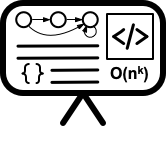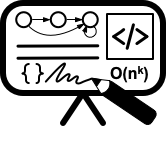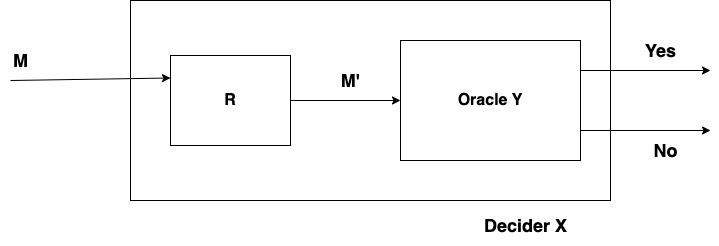# Lecture 22 - Decidability I

Date Pre-lecture slides Post-lecture scribbles Lecture recording
November 14 2023## Notes

#### Decidability

Decidability usually refers to the concept of whether a problem can be solved algorithmically or not. Specifically, decidability deals with the question of whether there exists an algorithm that can correctly determine whether a given input satisfies a particular property or not.

#### Halting Problem

The halting problem is a classic example of an undecidable problem in computer science. It asks whether there exists an algorithm that can determine, for any given program and input, whether the program will eventually halt (i.e., stop executing) or run forever.

The halting problem has important implications in theoretical computer science and the foundations of computation. It is a fundamental result that shows that there are certain problems that are inherently undecidable, meaning that there is no algorithm that can solve them for all cases.

#### Reduction

Problem X reduces to problem Y, if given a solution to Y, then it implies a solution for X. Namely, we can solve Y then we can solve X. We will done this by X => Y. This youtube video makes it easier to understand how reduction works.